Next: Phonon dispersions Up: Description of the program Previous: Description of the program

## Super-cell generation

The first step to calculate phonons in a crystal is to construct the super-cell, this can be done using the following setting:

LSUPER =.TRUE. (default)
NDIM = NX NY NZ (default: 1 1 1)
NTYPES = 2 (no default)

where NX, NY and NZ are positive integer numbers. NTYPES is the number of different atomic species (2 in the Stishovite example). PHON reads the file POSCAR which must contain a super-cell,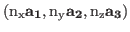(possibly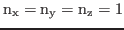) and generates the file SPOSCAR which contains the super-cell whose lattice vectors are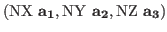, where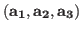are the lattice vectors of the primitive cell. Do not use NX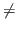NY if the crystal has a symmetry operation which connects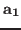with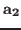. For face-centred-cubic (FCC) and body-centred-cubic (BCC) crystals do not use simple-cubic lattice vectors with 4 and 2 atoms in the primitive cell respectively, use proper FCC and BCC lattice vectors and one atom per primitive cell.

The program prints out a guess for the displacements needed to construct the full force field. These are written on the stout and in the file DISP. The size of the displacement can be changed setting the variable DISP:

DISP = 25 (default, corresponding to displacements of 0.04 Å).

In principle, a single off symmetry displacement could be enough to generate the full force field, as three linearly independent displacement may be generated from this using the symmetry operations of the crystal (this may not be true if the crystal has low symmetry). In practice, however, one would like to use displacements that alter as little as possible the symmetry of the crystal, because this minimises the computational effort in ab-initio calculations (maximising the number of symmetry operations in general reduces the number of k-points needed). For this reason, the displacements suggested by the program work on the assumption that the first displacement will be along the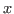-axis, and appropriate additional displacements along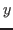and/or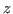are suggested if they are needed. The user may suggest his own first displacement using the variable: DXSTART:

DXSTART = xstart ystart zstart

To use central differences (eqn. 4 instead of eqn. 3), set the variable LCENTRAL = .T.Next: Phonon dispersions Up: Description of the program Previous: Description of the program
Dario Alfe` 2012-02-20